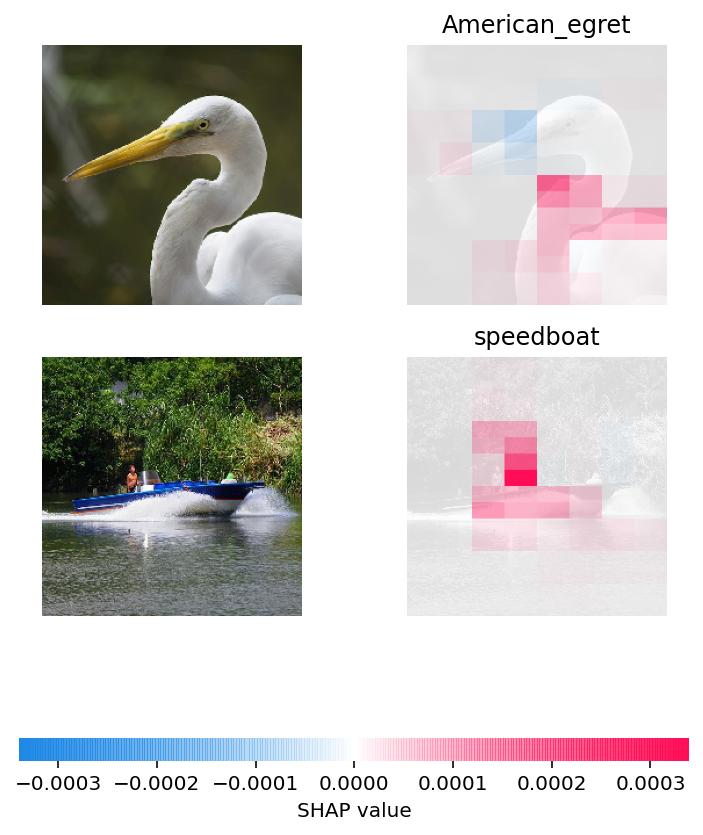# Multi-class ResNet50 on ImageNet (TensorFlow)

:

from tensorflow.keras.applications.resnet50 import ResNet50, preprocess_input
import json
import shap
import tensorflow as tf

# load pre-trained model and choose two images to explain
model = ResNet50(weights='imagenet')
def f(X):
tmp = X.copy()
preprocess_input(tmp)
return model(tmp)
X, y = shap.datasets.imagenet50()

# load the ImageNet class names as a vectorized mapping function from ids to names
url = "https://s3.amazonaws.com/deep-learning-models/image-models/imagenet_class_index.json"
with open(shap.datasets.cache(url)) as file:
class_names = [v for v in json.load(file).values()]

# define a masker that is used to mask out partitions of the input image, this one uses a blurred background

# By default the Partition explainer is used for all  partition explainer

explainers.Partition is still in an alpha state, so use with caution...

Partition explainer: 3it [00:18,  6.13s/it]